Question

# Q2) All sub problems are related. Show all steps for full credit. Let U and V...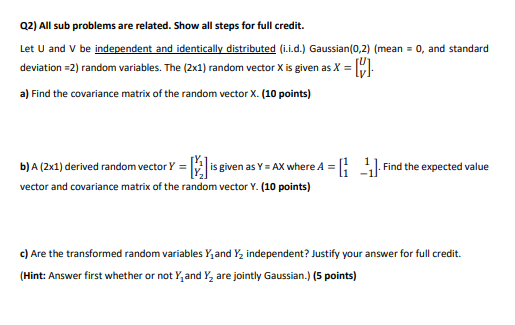Q2) All sub problems are related. Show all steps for full credit. Let U and V be independent and identically distributed (i.i.d.) Gaussian(0,2) (mean = 0, and standard deviation 2) random variables. The (2x1) random vector X is given as X = II a) Find the covariance matrix of the random vector X. (10 points) . Find the expected value b) A (2x1) derived random vector Y = 2 is given as Y = AX where A = [1 vector and covariance matrix of the random vector Y. (10 points) c) Are the transformed random variables Y and Y2 independent? Justify your answer for full credit. (Hint: Answer first whether or not Y, and Y, are jointly Gaussian.) (5 points)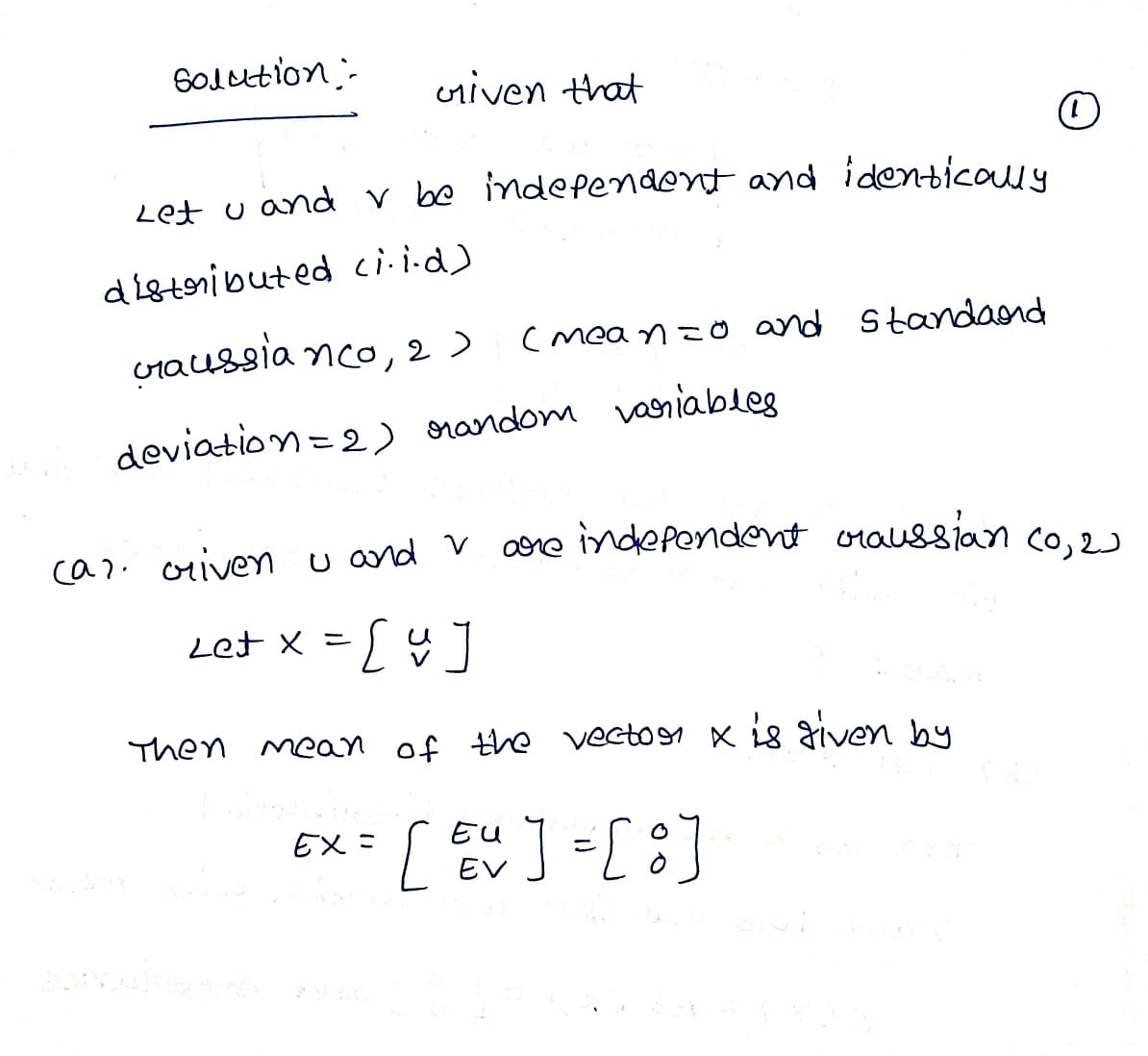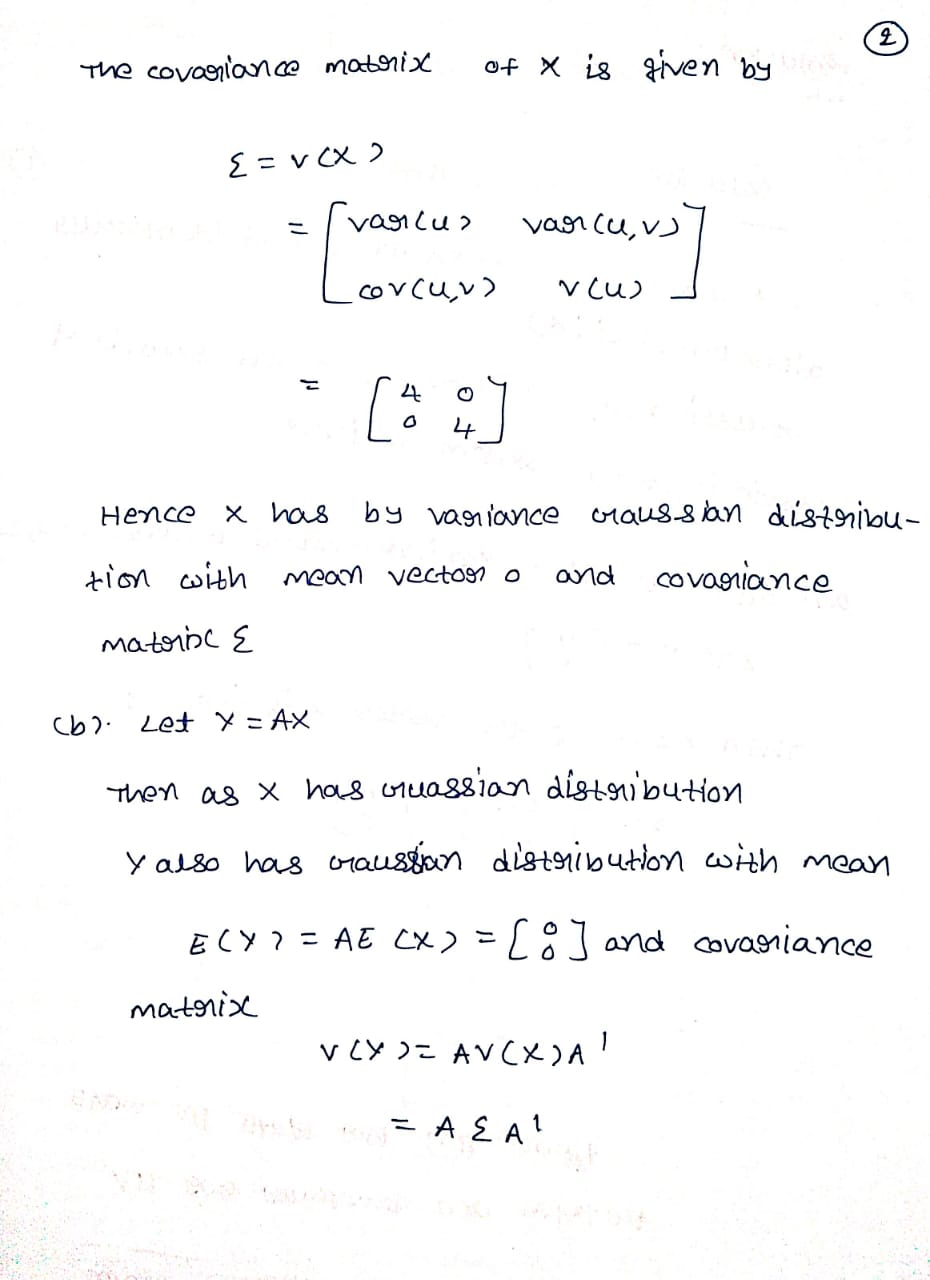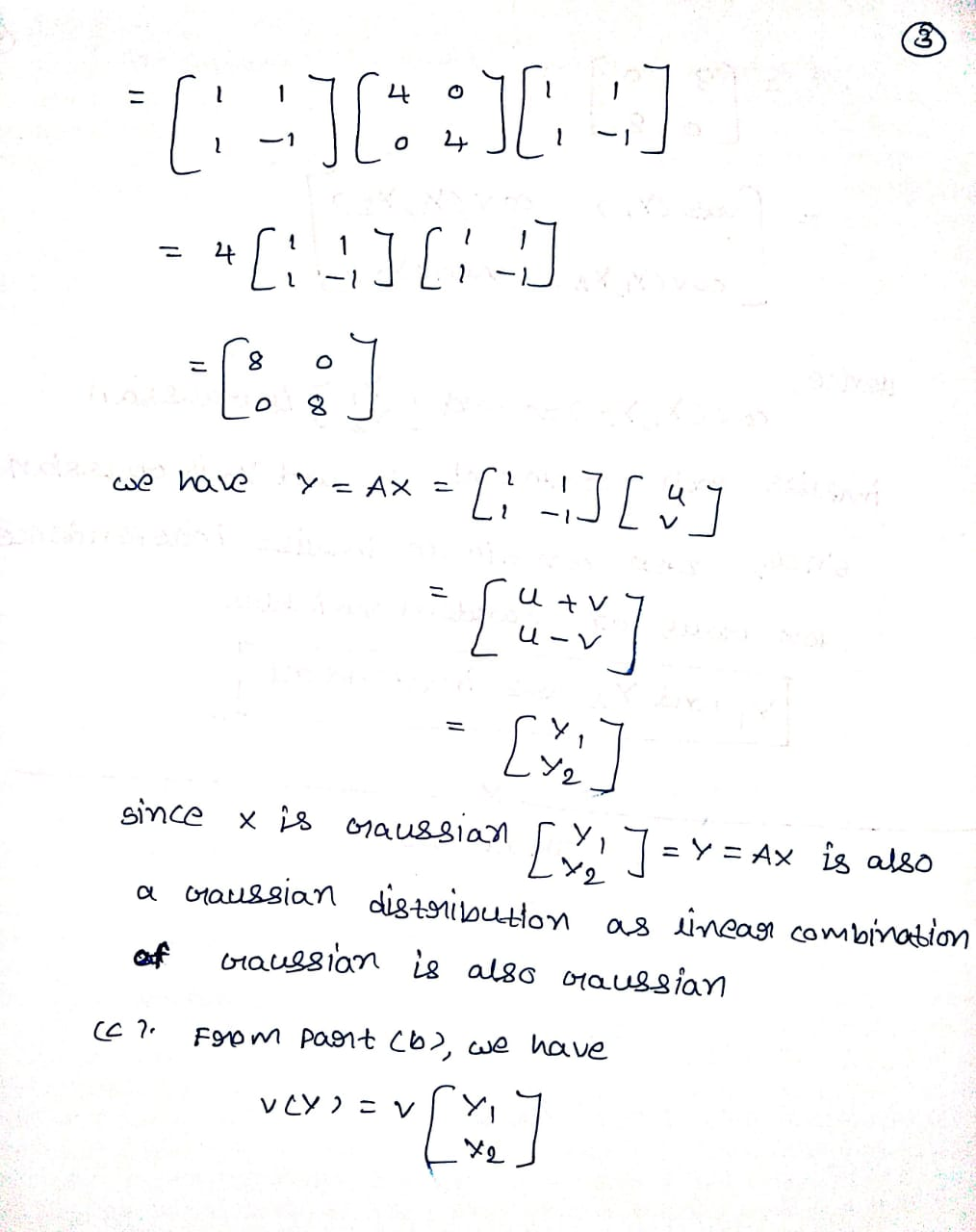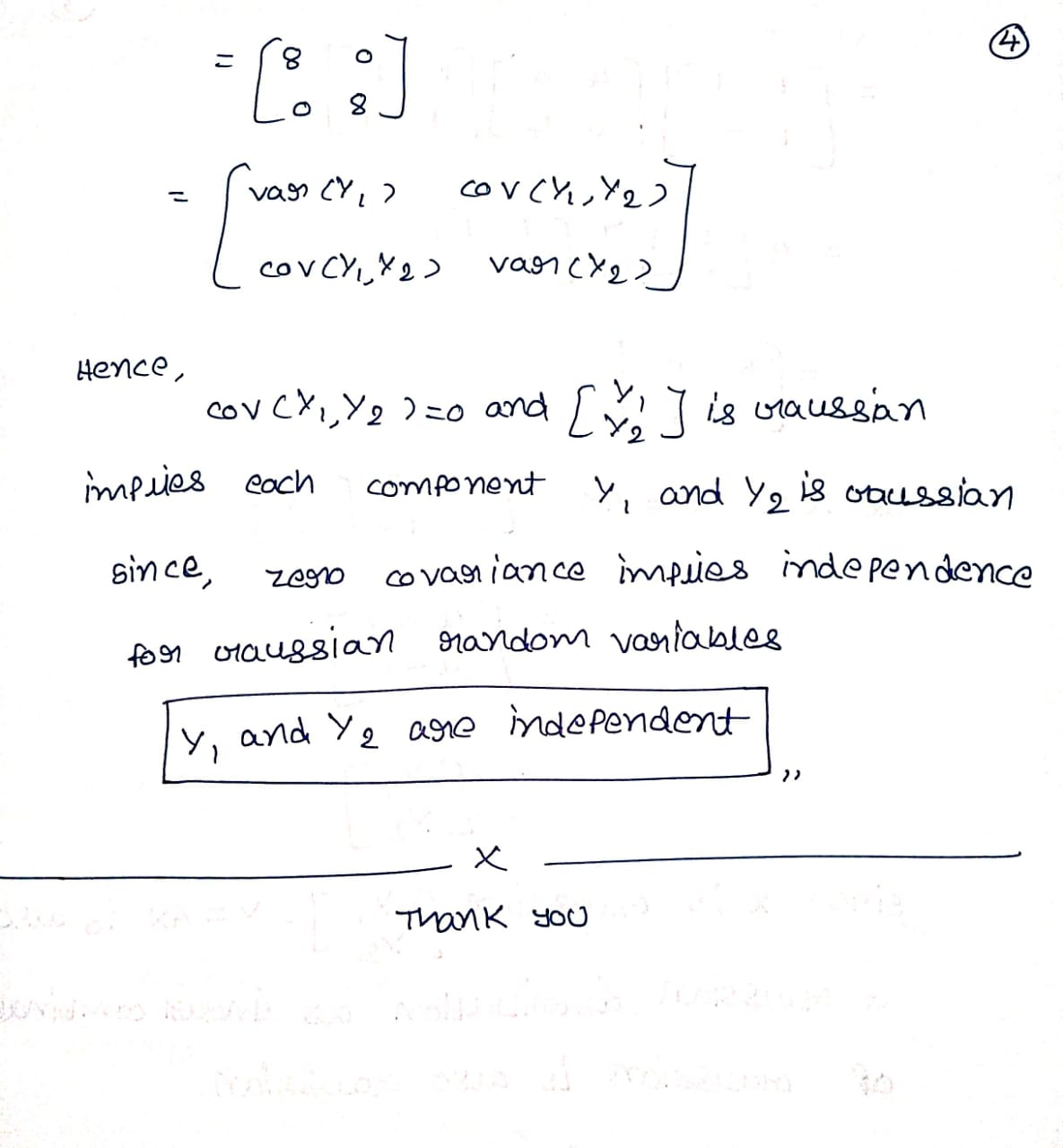#### Earn Coins

Coins can be redeemed for fabulous gifts.

Similar Homework Help Questions
• ### 1. (20 points) Let X (Xi, X, Xs) be a real random vector, where X, are identically dis- tributed and independent (i...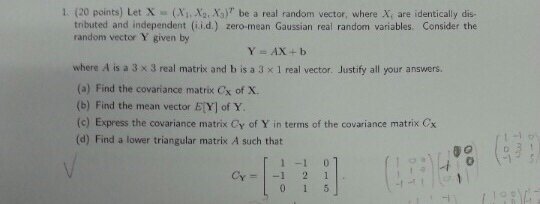1. (20 points) Let X (Xi, X, Xs) be a real random vector, where X, are identically dis- tributed and independent (ii.d.) zero-mean Gaussian real random variables. Consider the random vector Y given by where A is a 3 x 3 real matrix and b is a 3 x 1 real vector. Justify all your answers. (a) Find the covariance matrix Cx of x. (b) Find the mean vector EY] of Y (c) Express the covariance matrix Cy of Y...

• ### II. Derivations (You must show all your work for full credit.) i. Given the model y=XB+ɛ,...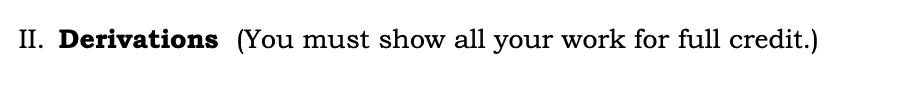II. Derivations (You must show all your work for full credit.) i. Given the model y=XB+ɛ, derive the least squares estimate for ß? (10 points) ii. Show that B=(x+x)"x"y is an unbiased estimate for B.(10 points) ii. Given vlə) = E[(@–B\–B)], derive the variance- covariance matrix for the least squares estimator (10 points). iv. Given the model y=XB+ɛ, the transformation matrix T, and TTT=22-1, derive the GLS estimator (10 points).

• ### Show all work and use correct notation for full credit. Stokes' Theorem: Let S be an...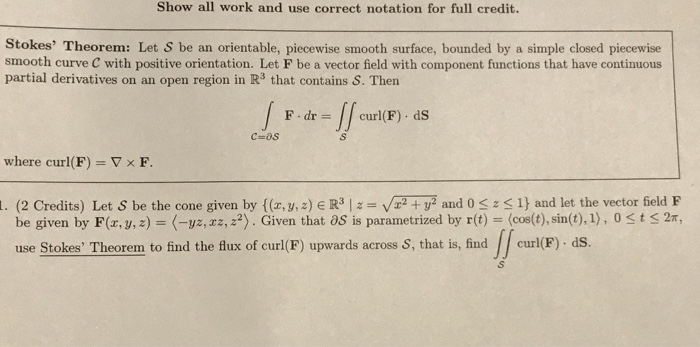Show all work and use correct notation for full credit. Stokes' Theorem: Let S be an orientable, piecewise smooth surface, bounded by a simple closed piecewise smooth curve C with positive orientation. Let F be a vector field with component functions that have continuous partial derivatives on an open region in R3 that contains S. Then | | curl(F) . ds F-dr = where curl(F) = ▽ × F. (2 Credits) Let S be the cone given by {(z, y,...

• ### The following problems should be turned in for credit. Show all of your work for full...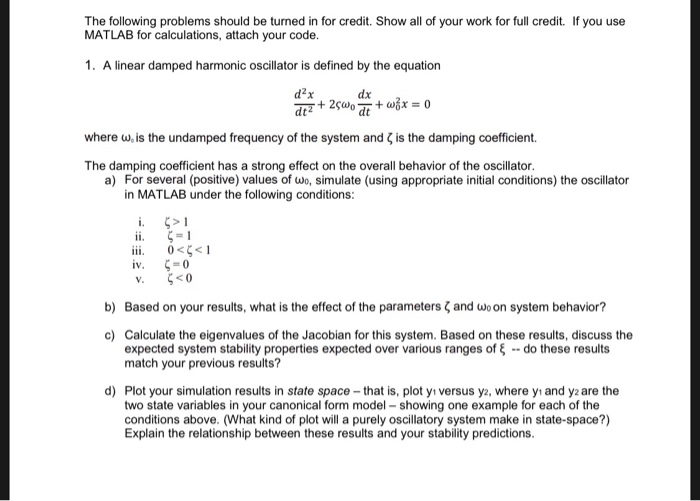The following problems should be turned in for credit. Show all of your work for full credit. If you use MATLAB for calculations, attach your code. 1. A linear damped harmonic oscillator is defined by the equation drz+ 25W + w x = 0 where w, is the undamped frequency of the system and is the damping coefficient. The damping coefficient has a strong effect on the overall behavior of the oscillator. a) For several (positive) values of wo, simulate...

• ### All work must be shown. That is, you need to show the steps and calculations you used to arrive at every answer for credit - For example, I need to see which two numbers you divided to arrive...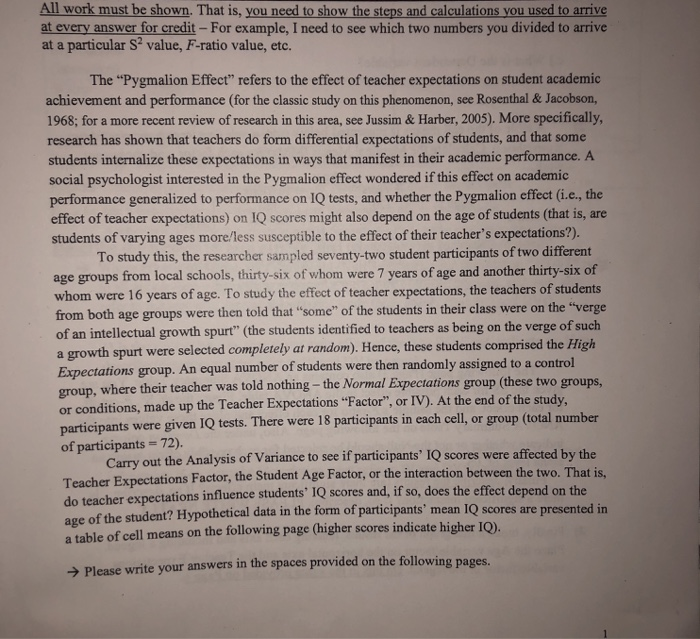All work must be shown. That is, you need to show the steps and calculations you used to arrive at every answer for credit - For example, I need to see which two numbers you divided to arrive at a particular S? value, F-ratio value, etc. The "Pygmalion Effect" refers to the effect of teacher expectations on student academic achievement and performance (for the classic study on this phenomenon, see Rosenthal & Jacobson, 1968; for a more recent review of...